5/19/2015

## Intro

In this chapter, we will discuss summarizing the distribution of numeric or quantitative variables.

Recall that numeric data is made up of measurements or numbers that describe our individuals with units.

Consider a data set that describes the rate of arrests per 100,000 residents for various types of crime in the 50 US states in 1973.

##            Murder Assault UrbanPop Rape
## Alabama      13.2     236       58 21.2
## Alaska       10.0     263       48 44.5
## Arizona       8.1     294       80 31.0
## Arkansas      8.8     190       50 19.5
## California    9.0     276       91 40.6
## Colorado      7.9     204       78 38.7

## Summarizing a Single Numeric Variable

When we look for numerical summaries of a quantitave variable, we are primarily interested in three things:

• Shape – what does the overall pattern of values look like?
• Measures of Center – what is a typical value?
• Measures of Spread – how far, on average, do individuals stray from the center?

These properties tell us about how the variable is distributed among our individuals. The distribution helps us answer questions like:

• What was the national average rate of arrests for murder in 1973?
• What percent of states arrested more people for assault per capita than Arizona?
• What was the arrest rate for rape in the middle 90% of states?

## Shape

To see the shape of the distribution, we typically rely on graphs. There are three main types of graphs for examining a single numeric variable:

• Histograms
• Steam and Leaf
• Boxplots

We will discuss the first two here and the third in a little while.

## Histograms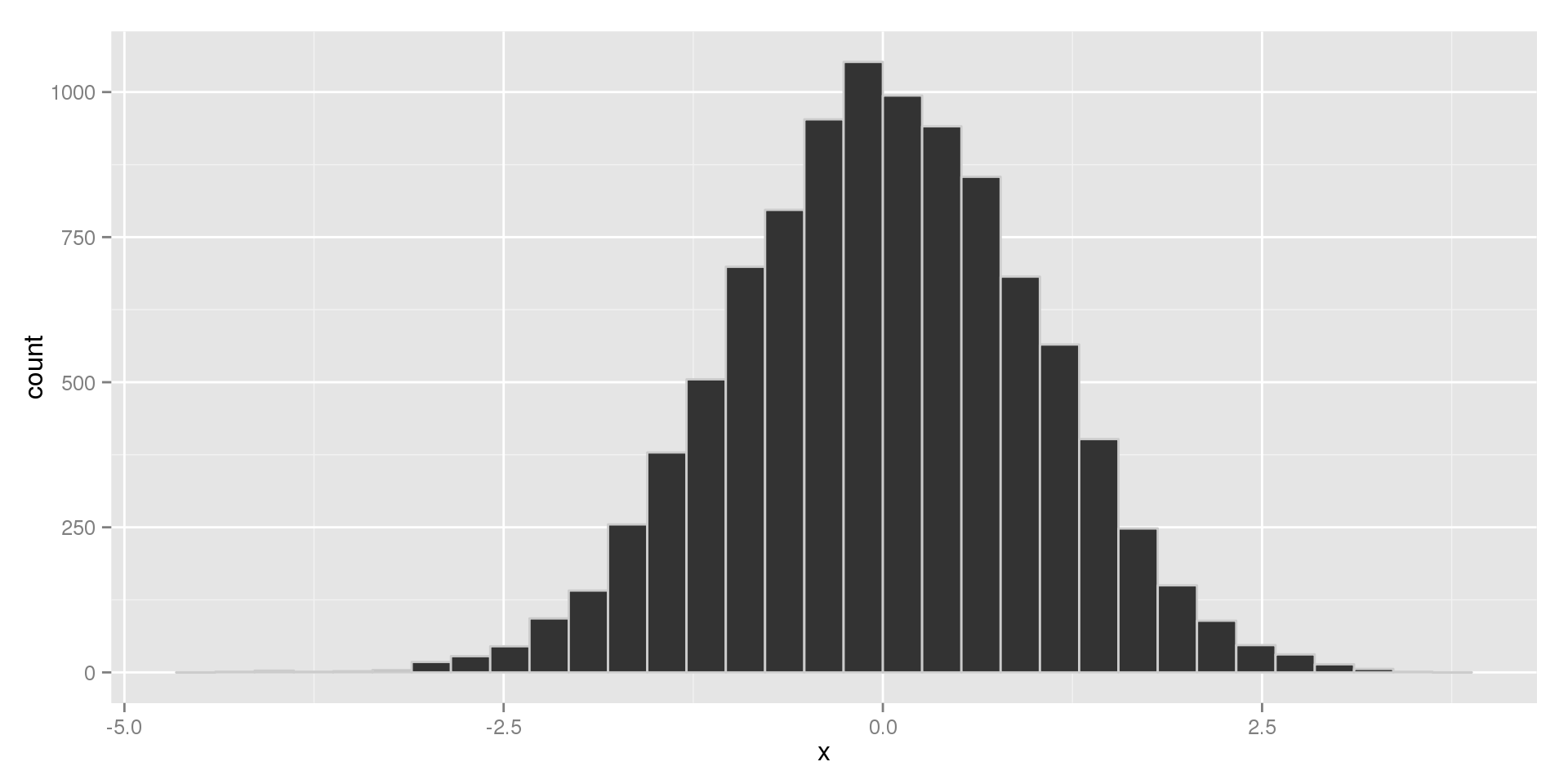## Histograms

Histograms are similar to bar charts, but the two are not interchangeable. Histograms are made by:

1. Creating an axis by dividing the variable into equal-sized "bins"
2. Drawing a bar in each bin whose height is given by the frequency of observations in that bin.

What do we look for?

• Any peaks in the distribution. The peaks are called modes.
• Symmetry – is the distribution symmetric around the center, or is it skewed to one side?
• Outliers – are there any unusual observations that are far away from the rest?

## Modes

When we describe a distribution by its modes, we use the terms:

• Unimodal if there is one peak
• Bimodal if there are two
• Multimodal or polymodal if there are more than two
• Uniform if the distribution is flat

What do modes tell us?

• Usually, having multiple modes mean there are distinct groups in the data
• For example, if we are looking at a histogram of heights, two modes might suggest we have a mix of men and women or adults and children in our sample

## Unimodal Histogram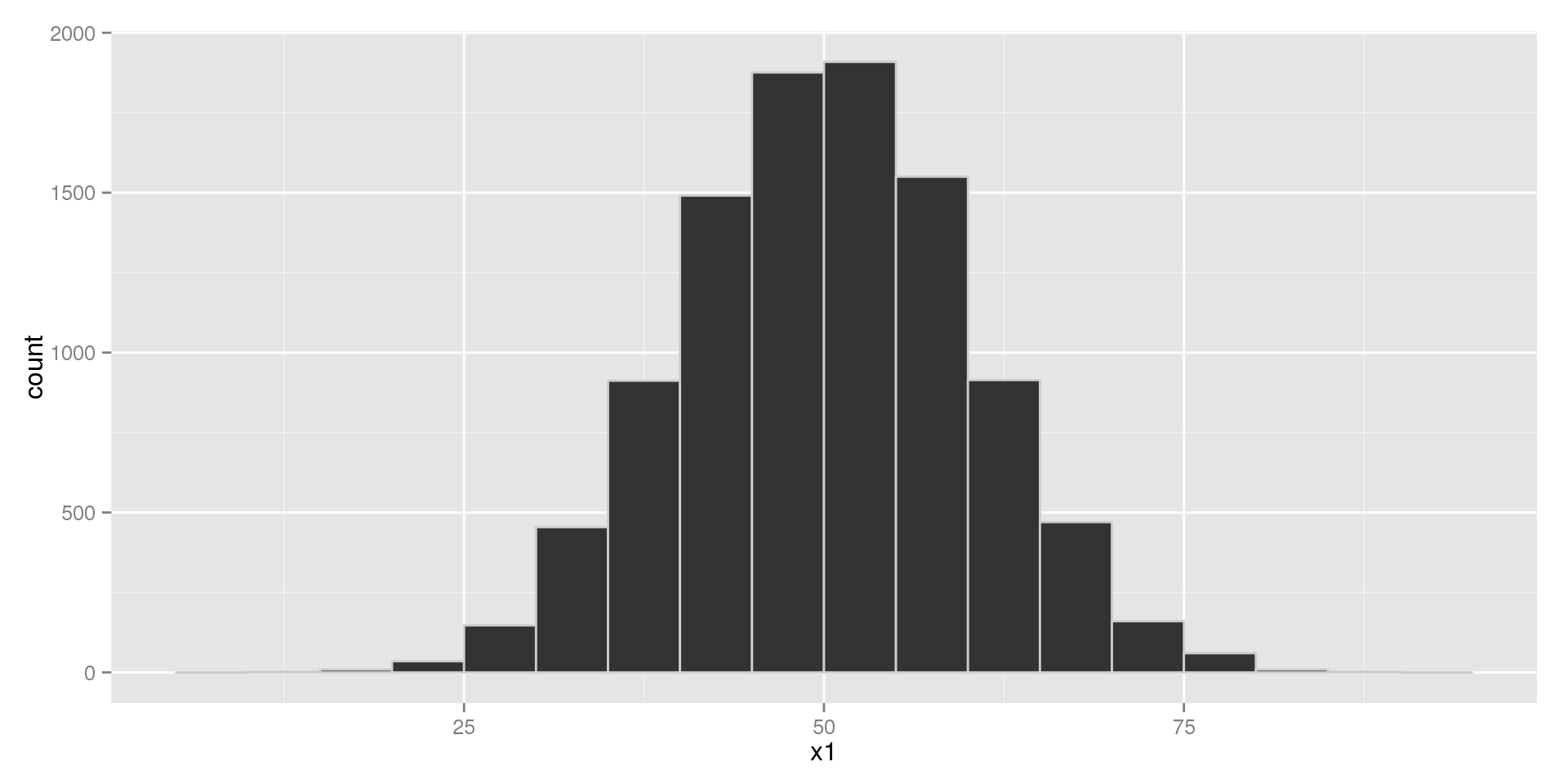## Bimodal Histogram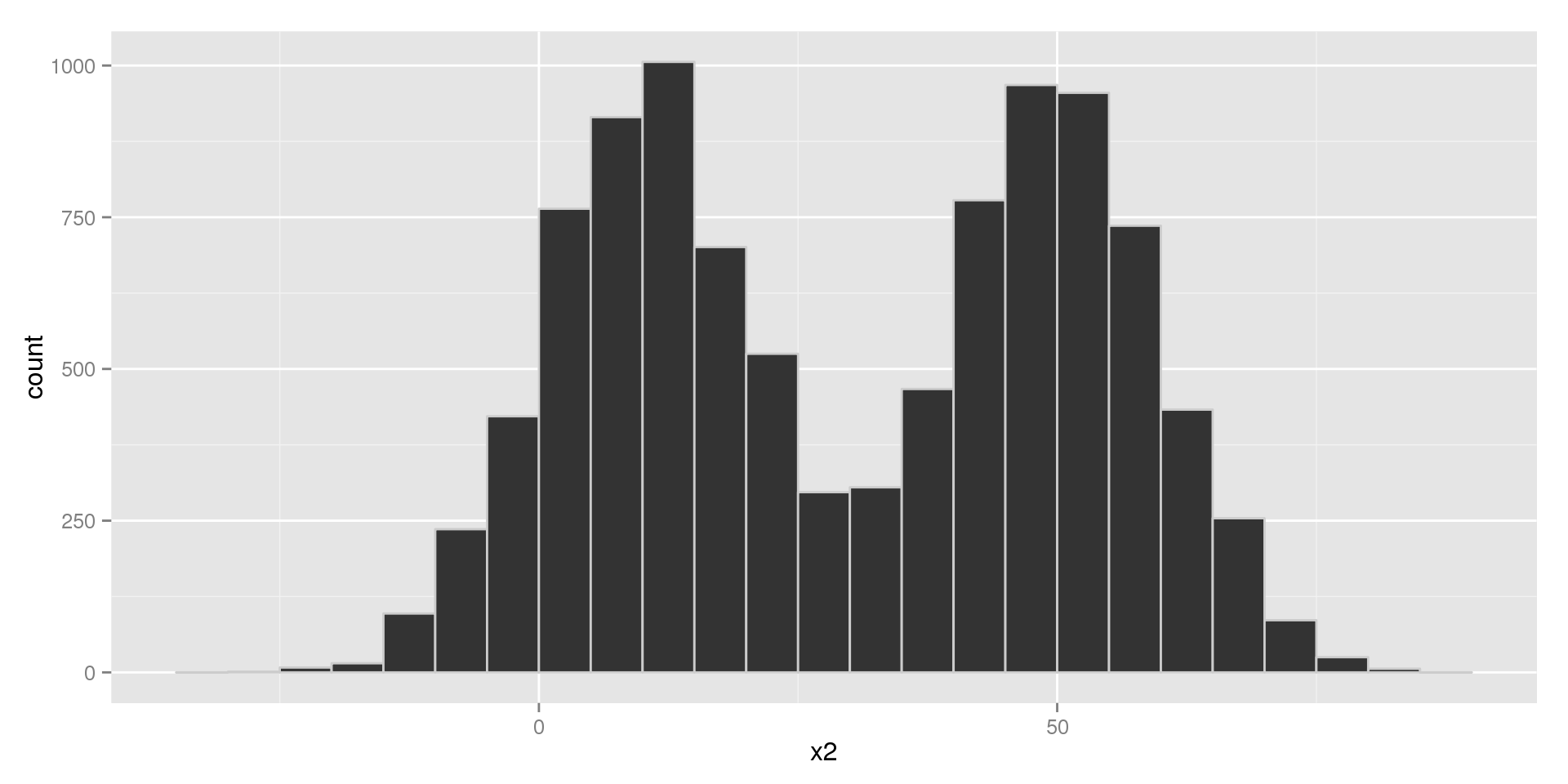## Multimodal Histogram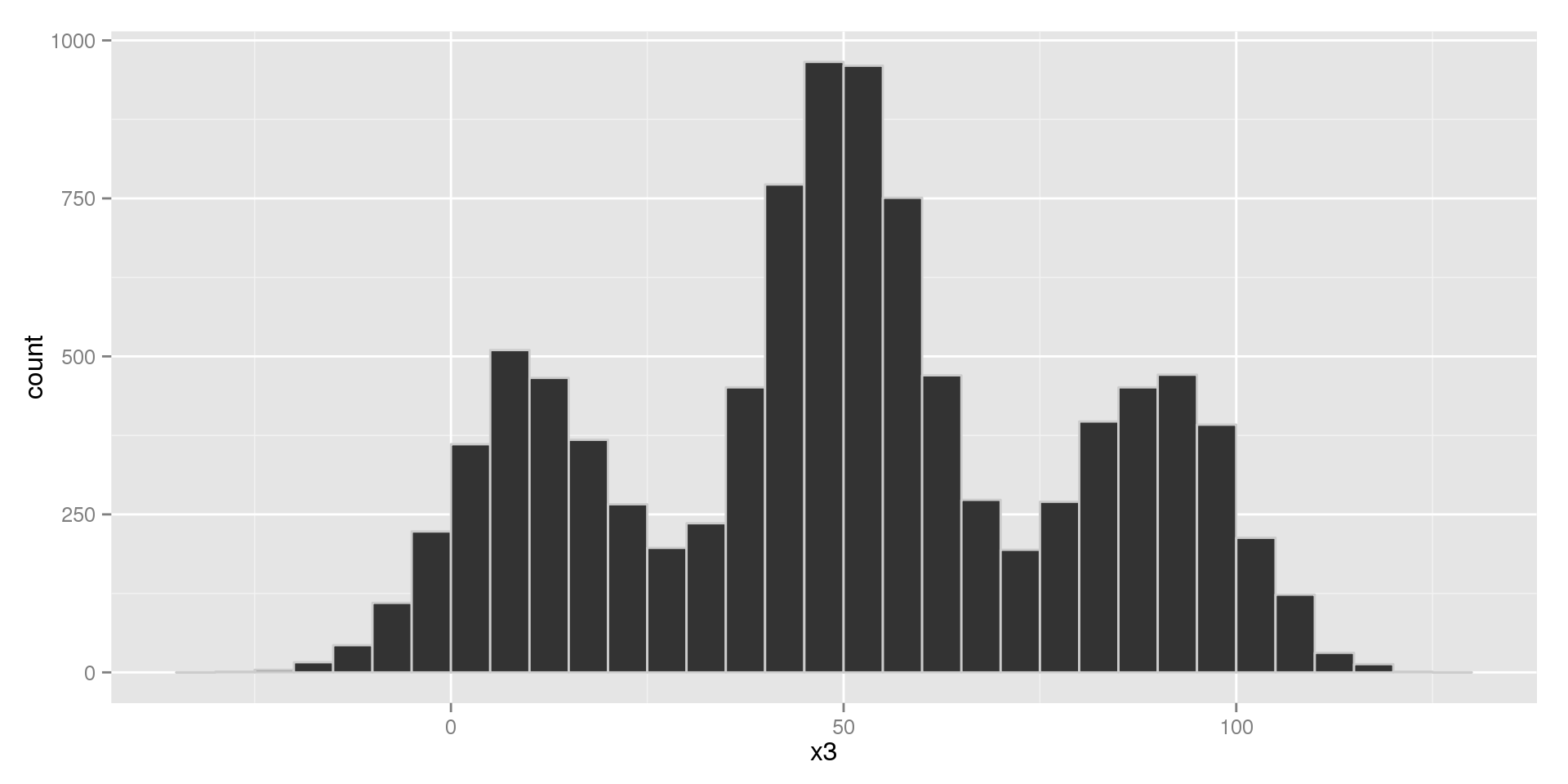## Uniform Histogram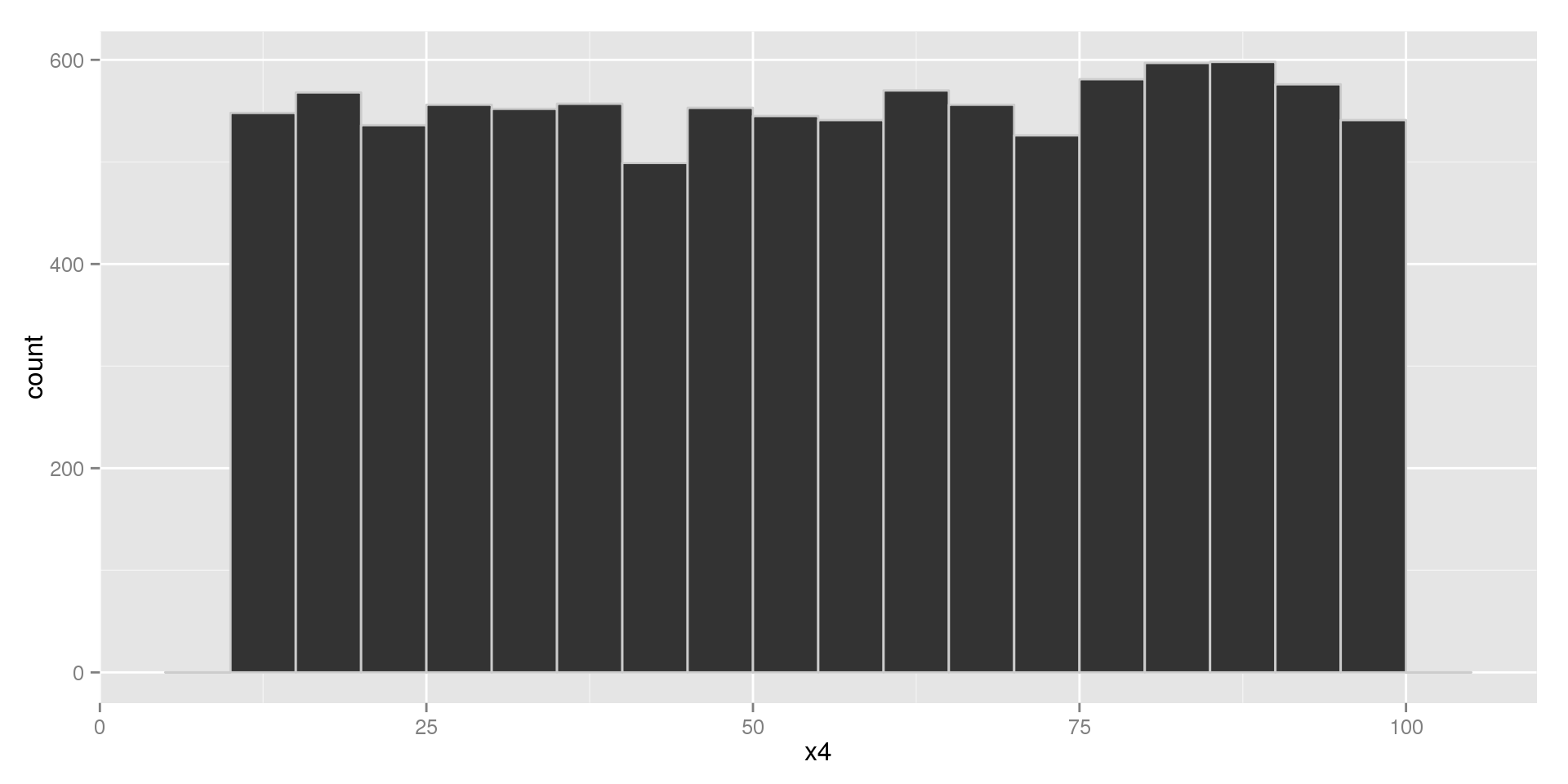## Symmetry

When we describe the symmetry of a distribution, we use the terms:

• Symmetric – when the distribution is symmetric about the center
• Right-Skewed – if there are some values higher than the majority
• Left-Skewed – if there are some values lower than the majority

We call the areas at either end the tails of the distribution. So, in terms of the tails:

• Symmetric: the right and left tails have equal length
• Right-Skewed: the right tail is longer
• Left-Skewed: the left tail is longer

Remember that when we talk about skew we're talking about the unusual points, not the majority.

## Symmetric Histogram## Right-Skewed Histogram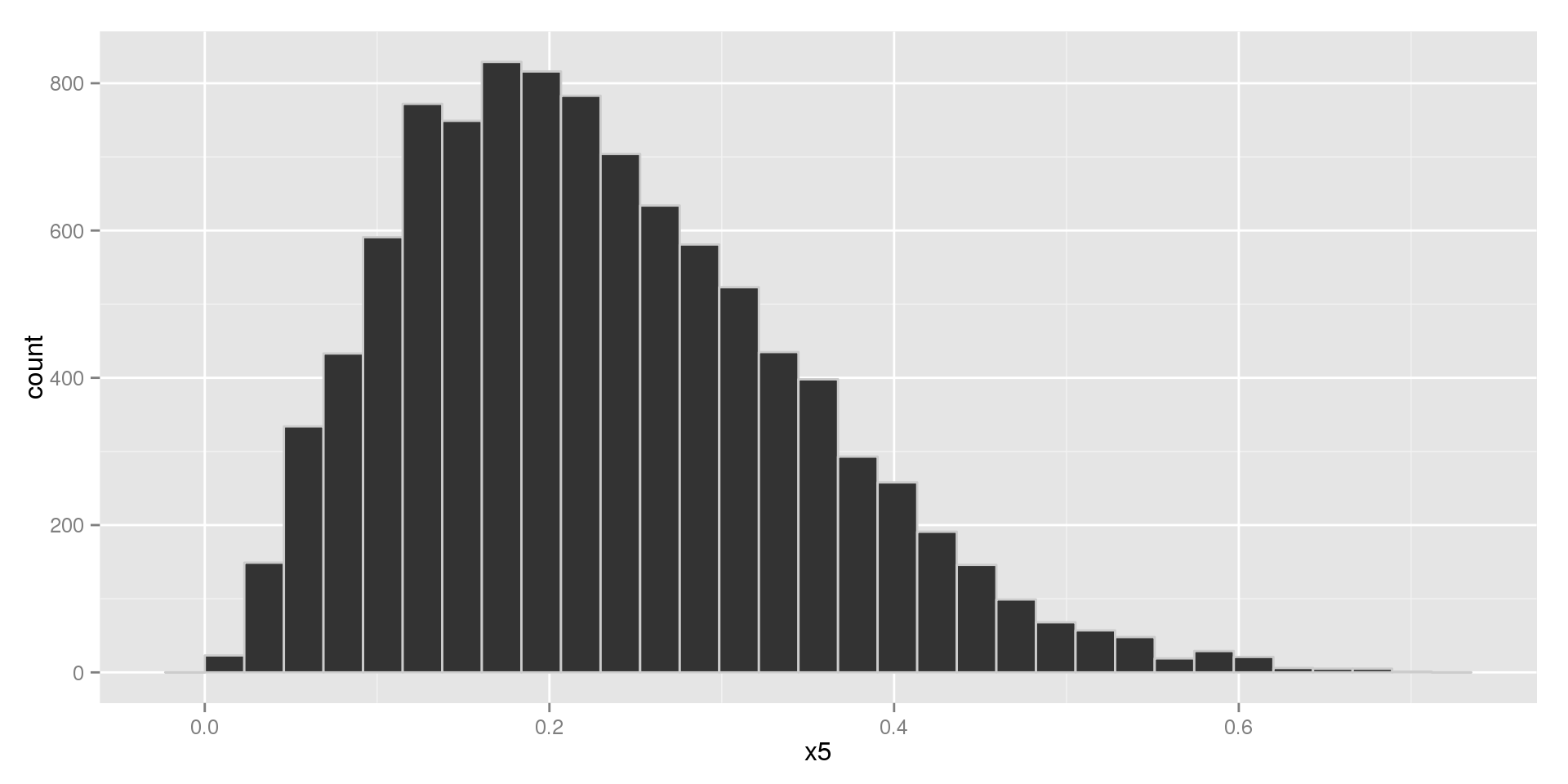## Left-Skewed Histogram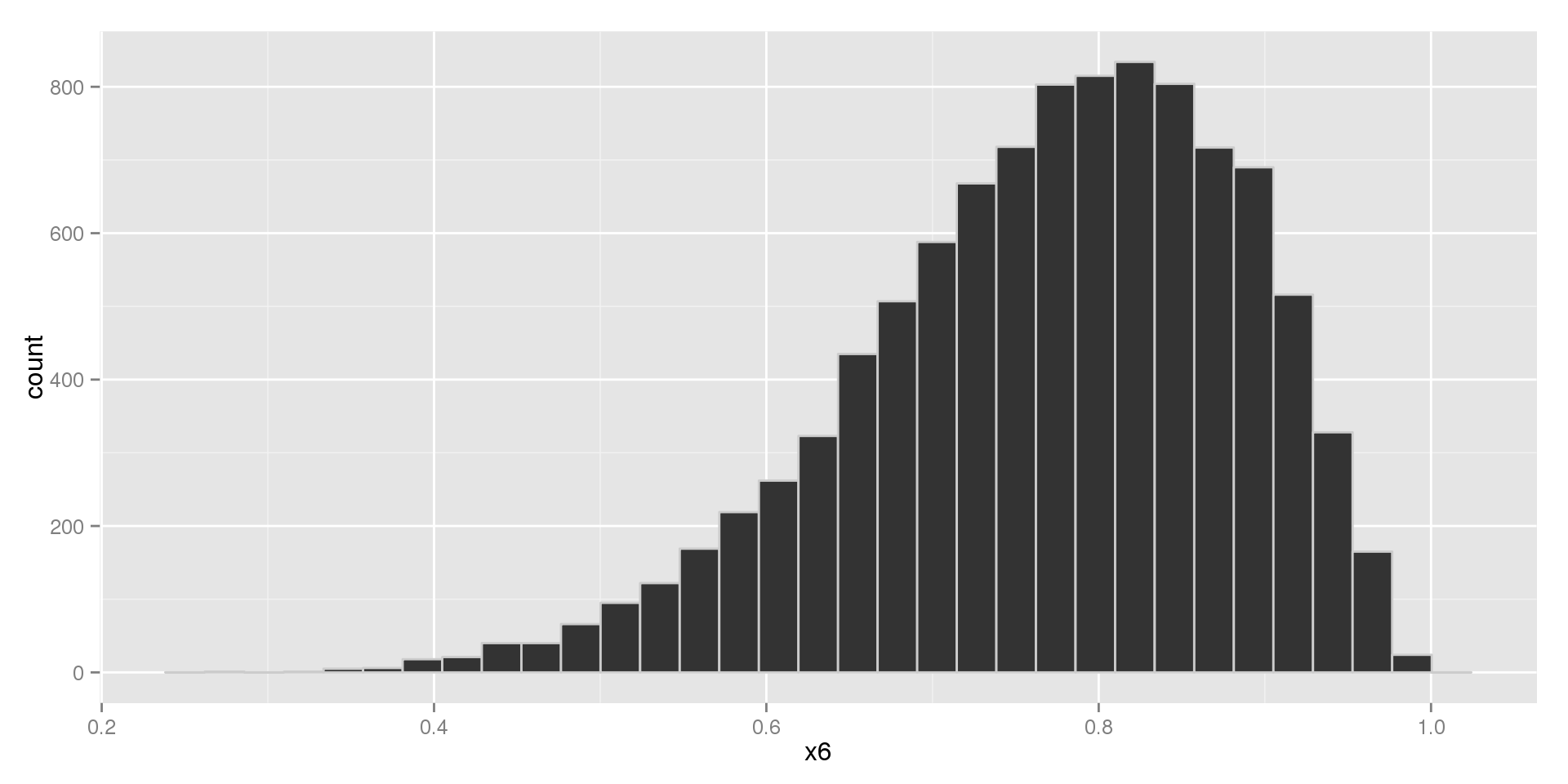## Outliers

We'll talk about outliers in more detail later, but generally:

• Outliers are any points that are substantially far away from the rest.

As a general rule-of-thumb,

• Outliers cause skew on whatever side they appear on.

## Histogram with Outliers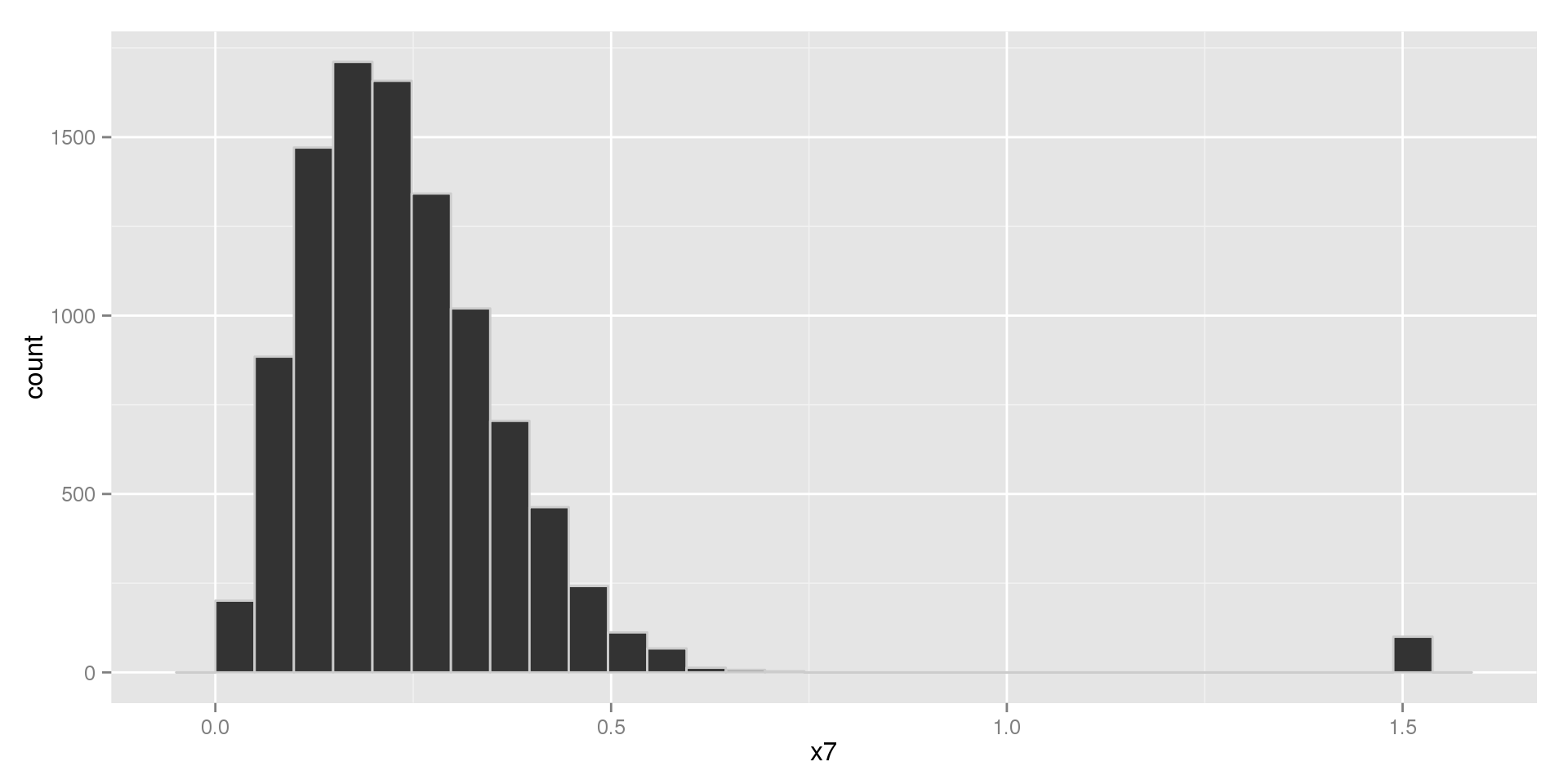## Cautions

Histograms are generally very good, but they have some things to be careful of:

• With smaller data sets they can be unstable (the shape can change drastically as you adjust the size of the bins). Interactive Example.
• It can be hard to find the true shape with small data sets
• Deciding on the bin width is a balancing act
• You lose information: we only know how many observations fall into a certain range, but we don't know anything about what they look like within the bin.

Describe the distribution of the variables from the US Arrests data set. The variables are:

• Murder: number of people arrested for murder (per 100,000 residents)
• Assault: number of people arrested for assault (per 100,000 residents)
• Rape: num of people arrested for rape (per 100,000 residents)
• UrbanPop: percent of population living in large cities

## Murder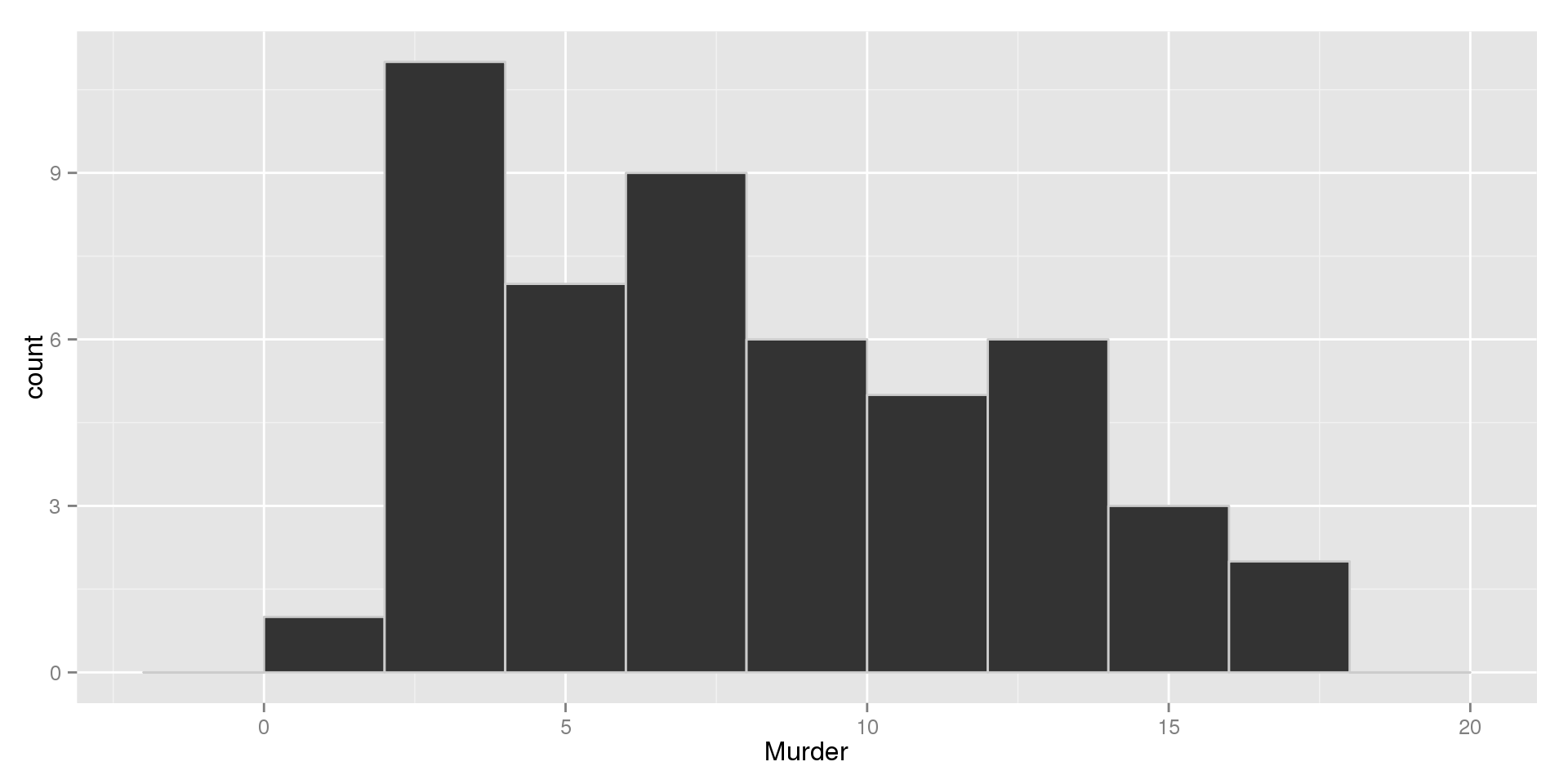## Assault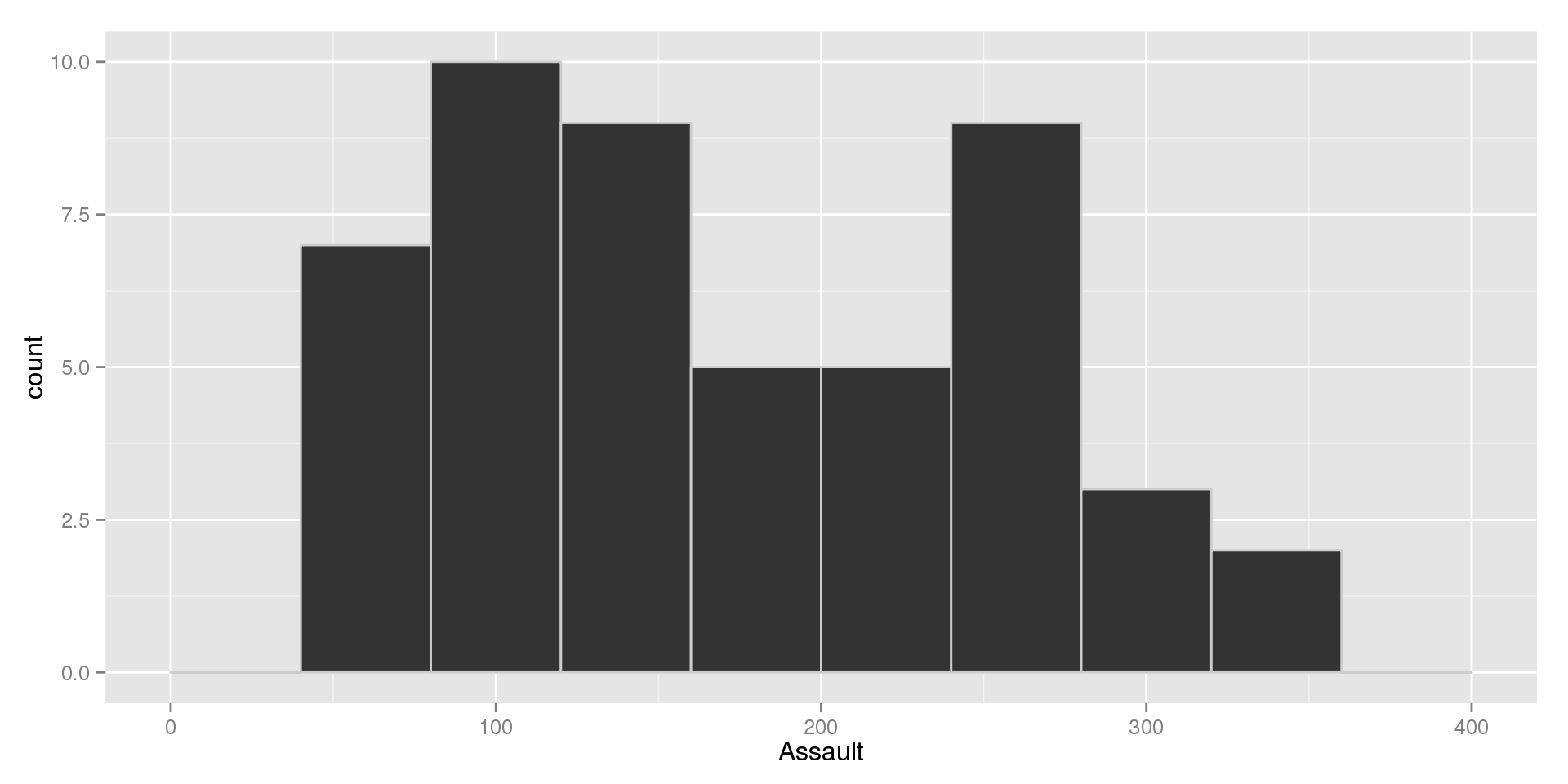## Rape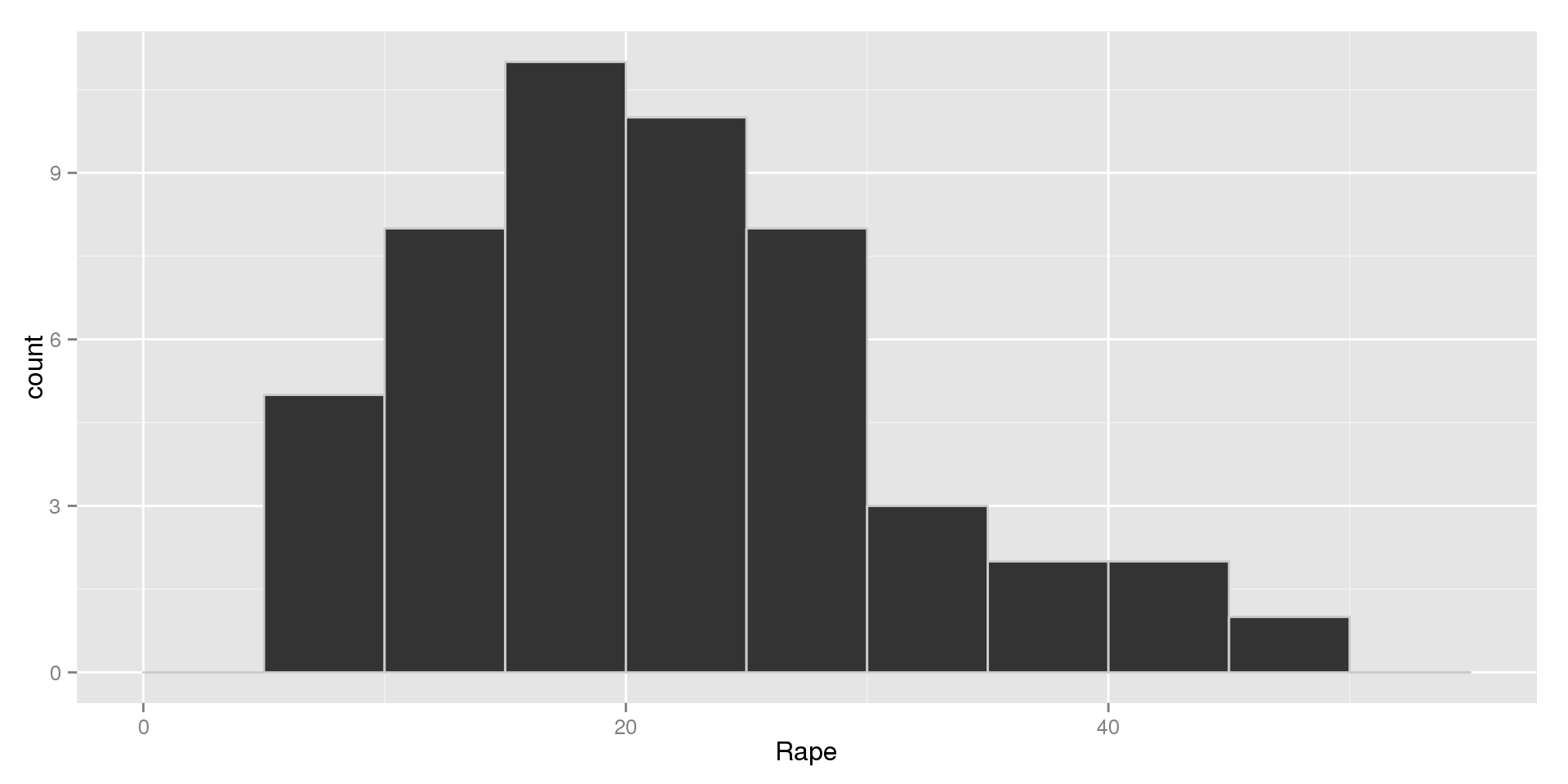## UrbanPop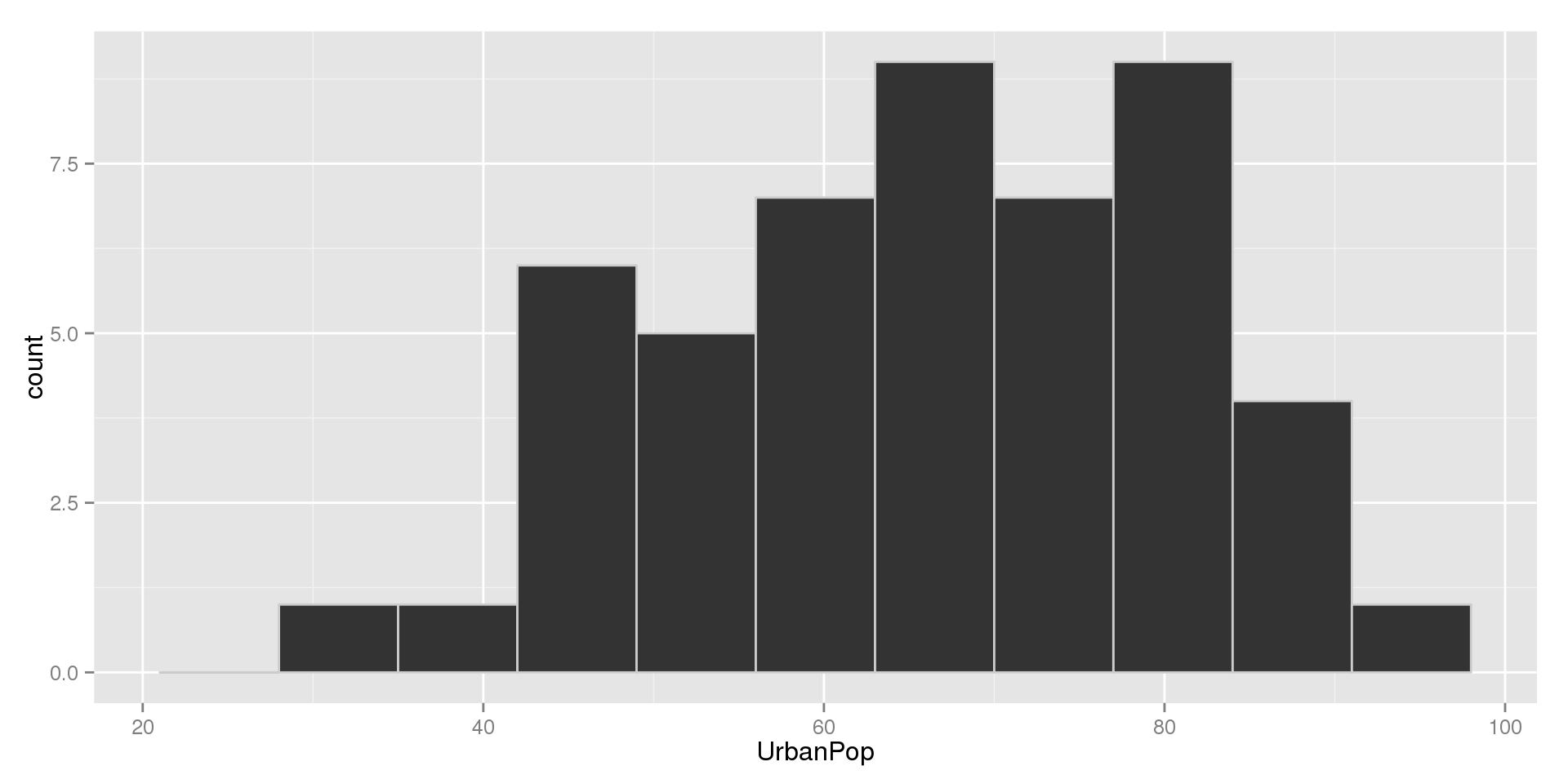## Stem-and-Leaf Plots

##
##   The decimal point is at the |
##
##   0 | 0000011111122222222233333333333444444444
##   0 | 55555666666666777788999999
##   1 | 000000001111111122223334
##   1 | 555689
##   2 | 134
##   2 | 9

## Stem-and-Leaf Plots

Stem-and-Leaf plots are similar to histograms, visually. We construct stem-and-leaf plots by:

• Breaking up each value at some decimal place (at the decimal point, at the ones place, at the tens place, etc)
• Grouping numbers that share the value to the left of that place and writing it as a "stem"
• Writing each value to the right of the chosen decimal place as a "leaf"
• The leaf should be a single digit, so round anything past the first digit to the right of the decimal place you've chosen.

Choosing the decimal place for the stem is similar to choosing the number of bins in a histogram: it's a balancing act.

## Stem-and-Leaf Plot: Tens Example

Consider the data set: $$5, 10, 11, 12, 12, 22$$. Almost all the values are in the double digits, so breaking them up at the tens place seems reasonable.

Our stem-and-leaf plot would be:

##
##   The decimal point is 1 digit(s) to the right of the |
##
##   0 | 5
##   1 | 0122
##   2 | 2

## Stem-and-Leaf Plot: Ones Example

Consider the data set: $$1.2, 1.3, 0.5, 0.6, 3.7, 10.2$$. The most interesting differences in the numbers are probably at the ones place.

##
##   The decimal point is at the |
##
##    0 | 56
##    1 | 23
##    2 |
##    3 | 7
##    4 |
##    5 |
##    6 |
##    7 |
##    8 |
##    9 |
##   10 | 2

## Stem-and-Leaf Plot: Hundreds place

What about the data set: $$320, 302, 102, 150, 175, 504, 40$$?

##
##   The decimal point is 2 digit(s) to the right of the |
##
##   0 | 4
##   1 | 058
##   2 |
##   3 | 02
##   4 |
##   5 | 0

## Stem-and-Leaf Notes

A couple important points:

• If your data doesn't have values for the stem you've chosen, include the stem but leave the leaf blank. We're looking for the shape of the distribution, so gaps in the data are just as important as the values themselves
• If you have a repeated value, make sure each one gets a leaf
• Stem-and-Leaf Plots allow us to completely reconstruct our data set from the plot, unlike histograms
• They are increasingly hard to read for large data sets

Generally, we might prefer stem-and-leaf plots to histograms for small data sets, but histograms for larger sets.

## Measures of Center and Spread

There are many techniques to measure the center and spread of a variable's distribution. Usually measures of center and spread go together based on how they work. In this class, we will focus on two pairs:

Robust Measures: these measures are very reliable, no matter the shape of the distribution

• Center: The Median
• Spread: The Interquartile Range (IQR)

For Symmetric Distributions: these measures have more useful properties if the distribution is symmetric

• Center: The Mean

## The Median

The Median:

• Is the point in the distribution of our variable that cuts the data in half.
• Divides the area of a histogram into two equal regions

Finding the Median:

• Start by sorting the data
• If $$n$$ is odd: count over $$\frac{n+1}{2}$$ from either end
• If $$n$$ is even: count over $$\frac{n}{2}$$ and $$\frac{n}{2} + 1$$ and average these values

Note:

• If a value is repeated, include it when finding the median.

## Finding the Median: $$n$$ is Odd

Consider the data set: $$1,\; 7,\; 5,\; 30,\; 25,\; 18,\; 12$$

• Sort: $$1,\; 5,\; 7,\; 12,\; 18,\; 25,\; 30$$
• Count over $$\frac{n + 1}{2} = \frac{7+1}{2} =\frac{8}{2} = 4$$
• $$1,\; 5,\; 7,\; \mathbf{12},\; 18,\; 25,\; 30$$
• The median is $$12$$.

## Finding the Median: $$n$$ is Even

Consider the data set: $$1,\; 7,\; 5,\; 18,\; 30,\; 25,\; 18,\; 12$$

• Sort: $$1,\; 5,\; 7,\; 12,\; 18,\; 18,\; 25,\; 30$$
• Count over $$\frac{n}{2} = \frac{8}{2} = 4$$
• $$1,\; 5,\; 7,\; \mathbf{12},\; 18,\; 18,\; 25,\; 30$$
• Count Over $$\frac{n}{2} + 1 = \frac{8}{2} + 1 =\ 4 + 1 = 5$$
• $$1,\; 5,\; 7,\; \mathbf{12},\; \mathbf{18},\; 18,\; 25,\; 30$$
• Average These Values: $$\frac{12 + 18}{2} = \frac{30}{2} = 15$$
• The median is $$15$$

## Visualization of the Median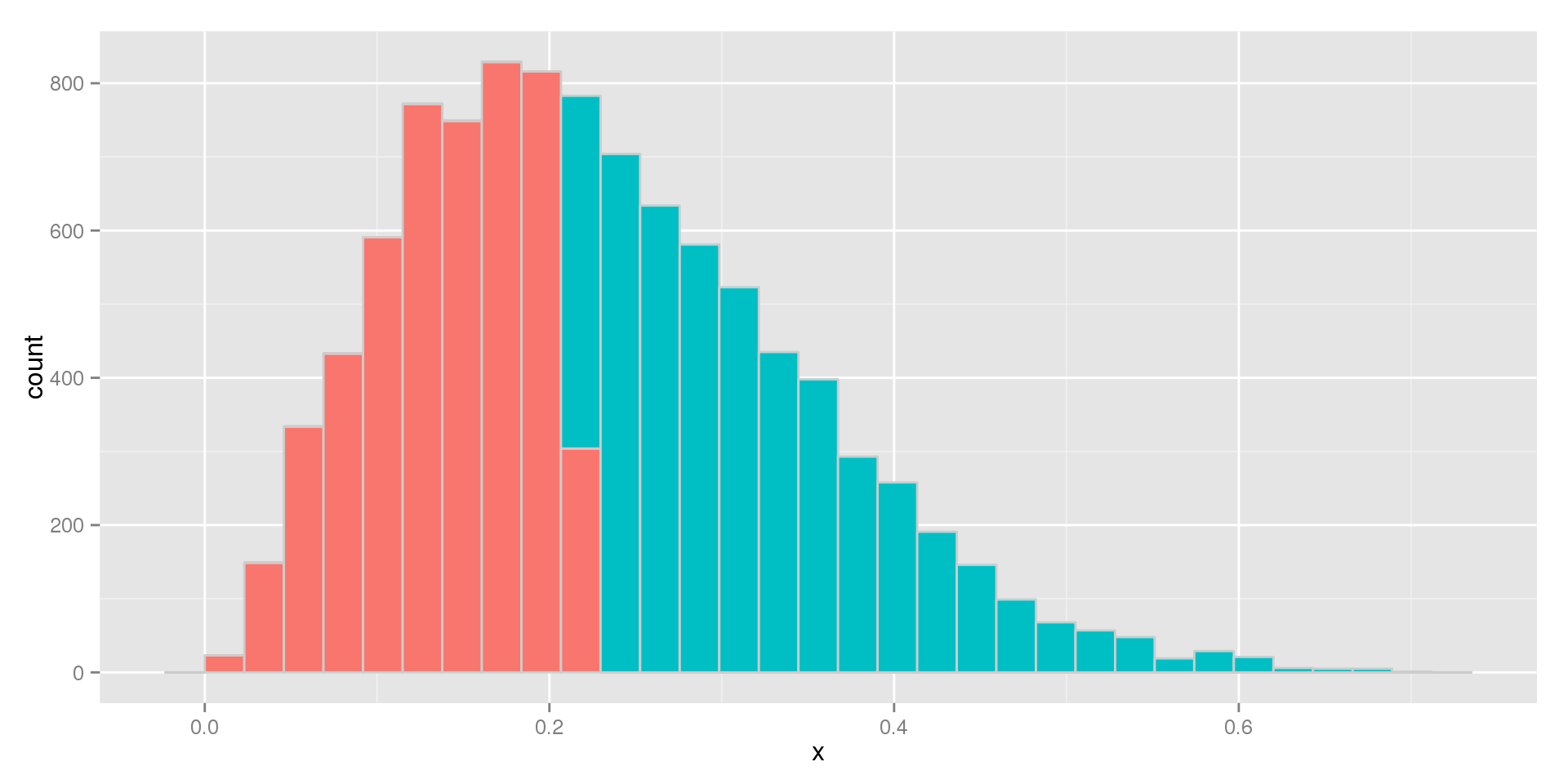## Measures of Spread: The Range

The most natural thing to look at to measure the spread of a sample might be to look at the overall range of the data.

• The range is the overall spread of the data
• $$range = max - min$$

Consider our data from earlier: $$1,\; 7,\; 5,\; 30,\; 25,\; 18,\; 12$$

• $$range = 30 - 1 = 29$$

What about the second set? $$1,\; 7,\; 5,\; 18,\; 30,\; 25,\; 18,\; 12$$

• $$range = 30 - 1 = 29$$

Note that adding a value in the middle of the distribution doesn't change the range.

## The Range (cont.)

But what happens if we have an outlier? $$1,\; 7,\; 5,\; 18,\; 60,\; 25,\; 18,\; 12$$

• $$range = 60 - 1 = 59$$

What does this mean?

• Most of the data is the same, but the range has doubled.
• Does this seem like a good property?
• In most circumstances, no.
• We need a measure of spread that is less sensitive to outliers.

## Measures of Spread: The Interquartile Range

Instead of looking at the extreme values in our distribution, we can look points closer to the center

After sorting our data, we divide it into fourths, based around the quartiles. These four groups are defined by five points, which make up the five number summary

• The Min: 0% of observations fall below this point
• The 1st Quartile ($$Q1$$): 25% of observations fall below this point
• The 2nd Quartile: aka The Median, 50% of observations fall below this point
• The 3rd Quartile ($$Q3$$): 75% of observations fall below this point
• The Max: 100% of observations fall below this point

## Finding the Quartiles:

We already know how to find three of them: the min, median, and max. So how can we find the 1st and 3rd quartiles?

• The Median divides the data into two equal halves
• The 1st Quartile is the median of the lower half
• The 3rd Quartile is the median of the upper half

Note:

• If $$n$$ is odd, include the median in both halves

## Finding the Quartiles: $$n$$ is Odd

$1,\; 7,\; 5,\; 30,\; 25,\; 18,\; 12$

• Sorted: $$1,\; 5,\; 7,\; 12,\; 18,\; 25,\; 30$$
• Recall that the median was $$12$$
• $$Q1$$ is the median of $$1,\; 5,\; 7,\; 12$$
• $$Q1 = \frac{5+7}{2} = \frac{12}{2} = 6$$
• $$Q3$$ is the median of $$12,\; 18,\; 25,\; 30$$
• $$Q3 = \frac{18 + 25}{2} = \frac{43}{2} = 21.5$$

So our Five Number Summary is:

• $$1,\; 6,\; 12,\; 21.5,\; 30$$

## Finding the Quartiles: $$n$$ is Even

$1,\; 7,\; 5,\; 18,\; 30,\; 25,\; 18,\; 12$

• Sorted: $$1,\; 5,\; 7,\; 12,\; 18,\; 18,\; 25,\; 30$$
• Recall that the median was $$15$$
• $$Q1$$ is the median of $$1,\; 5,\; 7,\; 12$$
• $$Q1 = \frac{5 + 7}{2} = \frac{12}{2} = 6$$
• $$Q3$$ is the median of $$18,\; 18,\; 25,\; 30$$
• $$Q3 = \frac{18 + 25}{2} = \frac{43}{2} = 21.5$$

So our Five Number Summary is:

• $$1,\; 6,\; 15,\; 21.5,\; 30$$

## The IQR

Now that we know what quartiles are, we can discuss the interquartile range (IQR). The IQR is defined as:

• $$IQR = Q3 - Q1$$
• Since 25% fall below $$Q1$$ and 75% below $$Q3$$, the IQR defines the range of the middle 50% of the data

Using either our previous examples:

• $$IQR = 21.5 - 6 = 15.6$$

## The IQR and Outliers

Using our data with an outlier from before: $$1,\; 7,\; 5,\; 18,\; 60,\; 25,\; 18,\; 12$$. Note that this is exactly the same as our most recent example, but the $$30$$ was changed to a $$60$$. The IQR in the previous example was $$15.6$$

• Sorted: $$1,\; 5,\; 7,\; 12,\; 18,\; 18,\; 25,\; 60$$
• Recall that the range was $$59$$, even though most of our values are fairly close together.
• $$Q1 = 6$$, $$Q3 = 21.5$$
• $$IQR = Q3 - Q1 = 21.5 - 6 = 15.6$$
• Despite the outlier, the IQR hasn't changed.
• We say that the IQR is robust to outliers

## Visualizing the IQR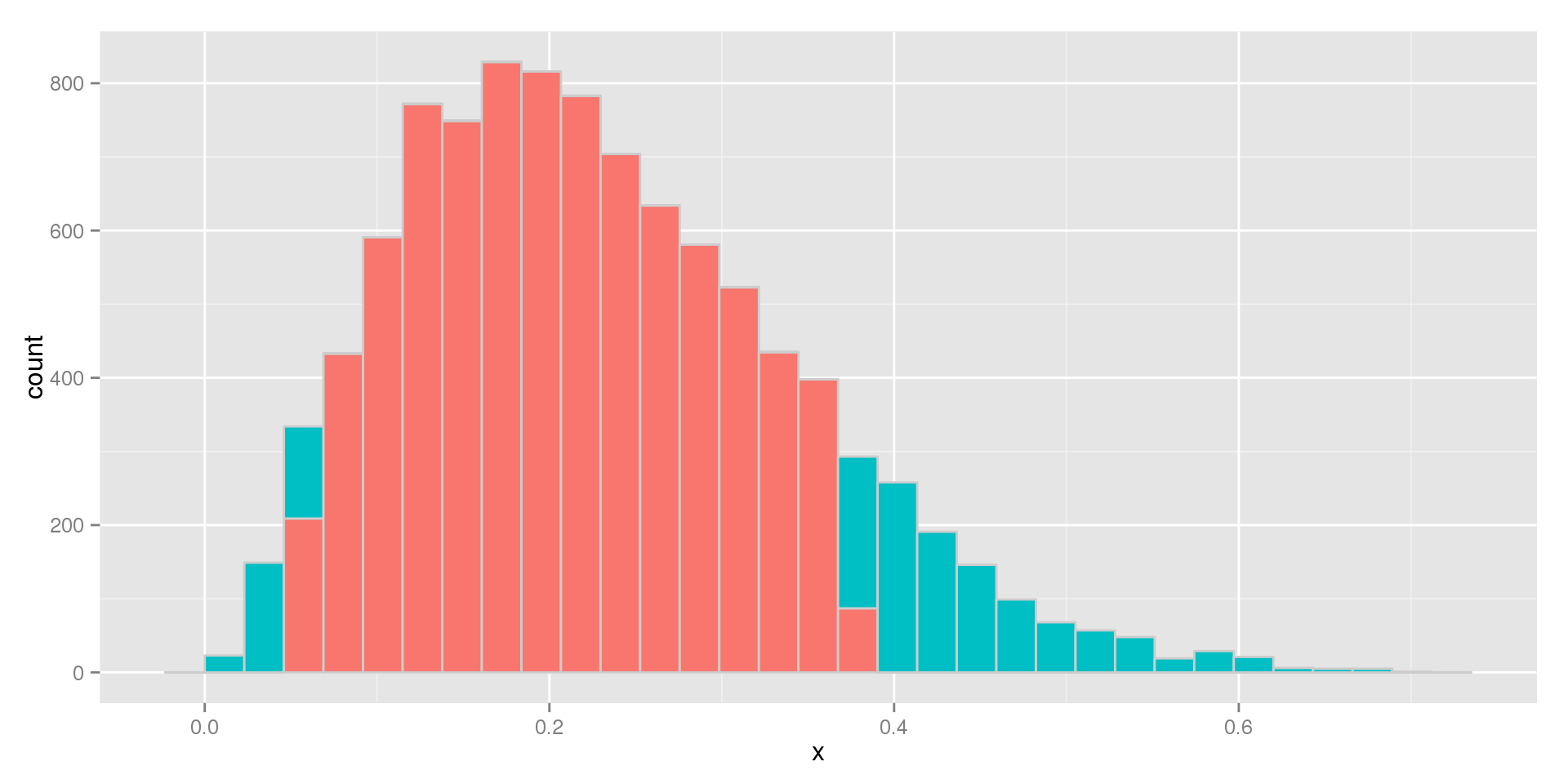## Defining Outliers

Giving a firm definition to outliers is a tricky thing. In different contexts, we may expect to see more extreme values than in others.

As a general rule:

• An outlier is an observation that falls more than 1.5 IQR's away from the median.

In our most recent example ($$1,\; 5,\; 7,\; 12,\; 18,\; 18,\; 25,\; 60$$), the median was $$15$$ and the IQR was $$15.6$$

• Any observation less than $$15 - (1.5 \times 15.6) = 15 - 23.4 = -8.4$$ would be an outlier
• Any observation greater than $$15 + (1.5 \times 15.6) = 15 + 23.4 = 38.4$$ would be an outlier

## Visualizing the 5 Number Summary

As an alternative to histograms and stem-and-leaf plots, which show the shape of a distribution in great detail, we can get a quick idea of the shape by plotting the five number summary.

Recall that the five number summary is made up of:

• The Min
• The First Quartile (Q1)
• The Median
• The Third Quartile (Q3)
• The Max

The graph of choice for the five number summary is the boxplot

## Boxplots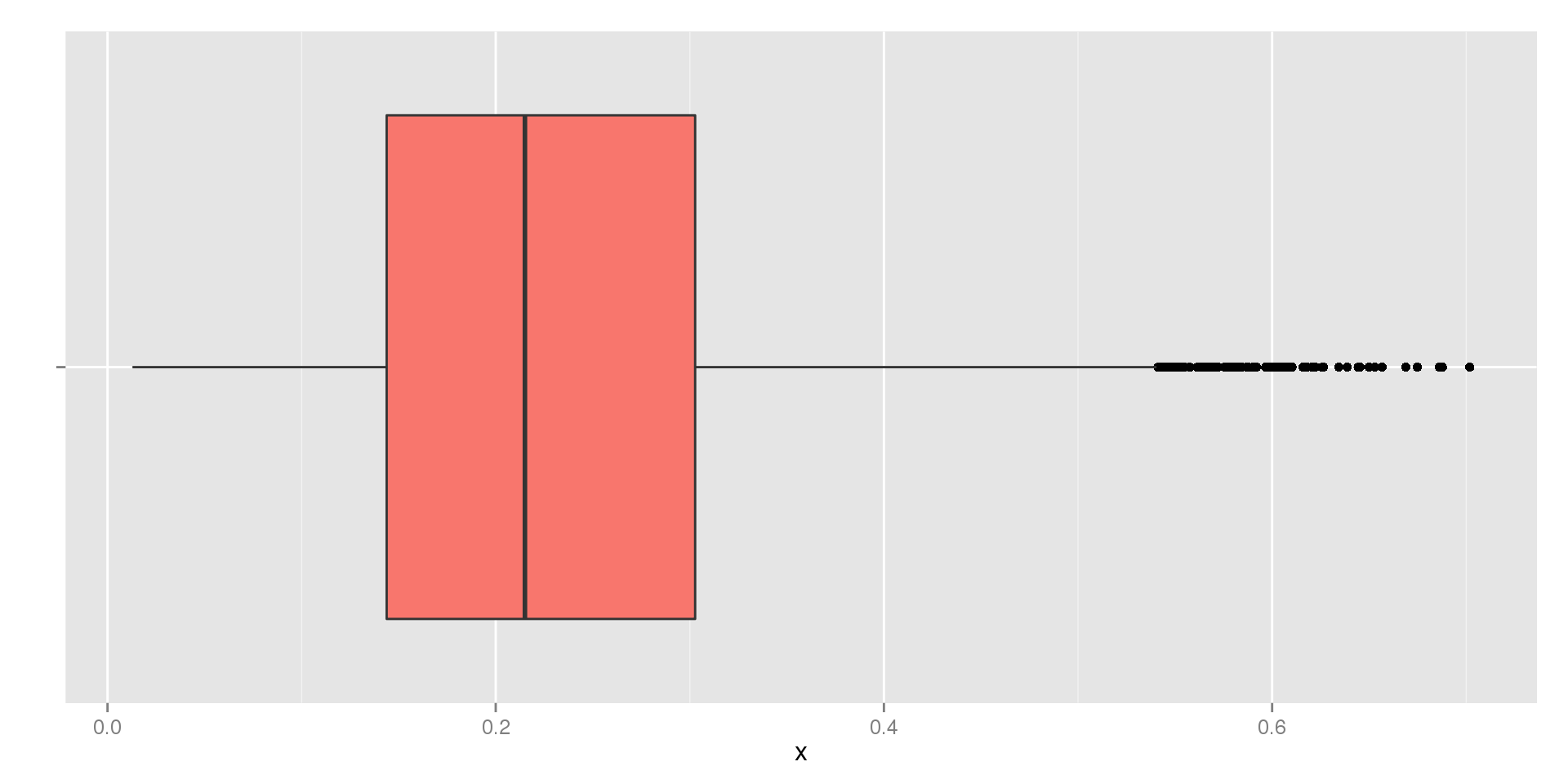## Constructing Boxplots

Note that boxplots can be drawn horizontally (as pictured in the previous slide) or vertically. Boxplots, also called box-and-whisker plots, have two major elements:

• The Box
• The Whiskers

Use one axis for the variable. If it is the horizontal axis, we call it a horizontal boxplot. If the variable's values are on the vertical axis, we call it a vertical boxplot.

To draw the box:

• The sides of the box that are perpendicular to the variable axis are drawn at Q1 and Q3, respectively
• The median is drawn in the box as a line perpendicular to the variable axis

## Drawing Boxplots (cont.)

The whiskers are a bit more complicated. In either case, they extend outward from the box on both sides along the variable axis. Two possibilities exist for each side.

If there are no outliers on that side:

• They extend from the box to the max or min

If there are outliers on that side:

• They extend out 1.5 IQRs from the mean
• Outliers are drawn as points past the end of the whisker

## Boxplot with Outliers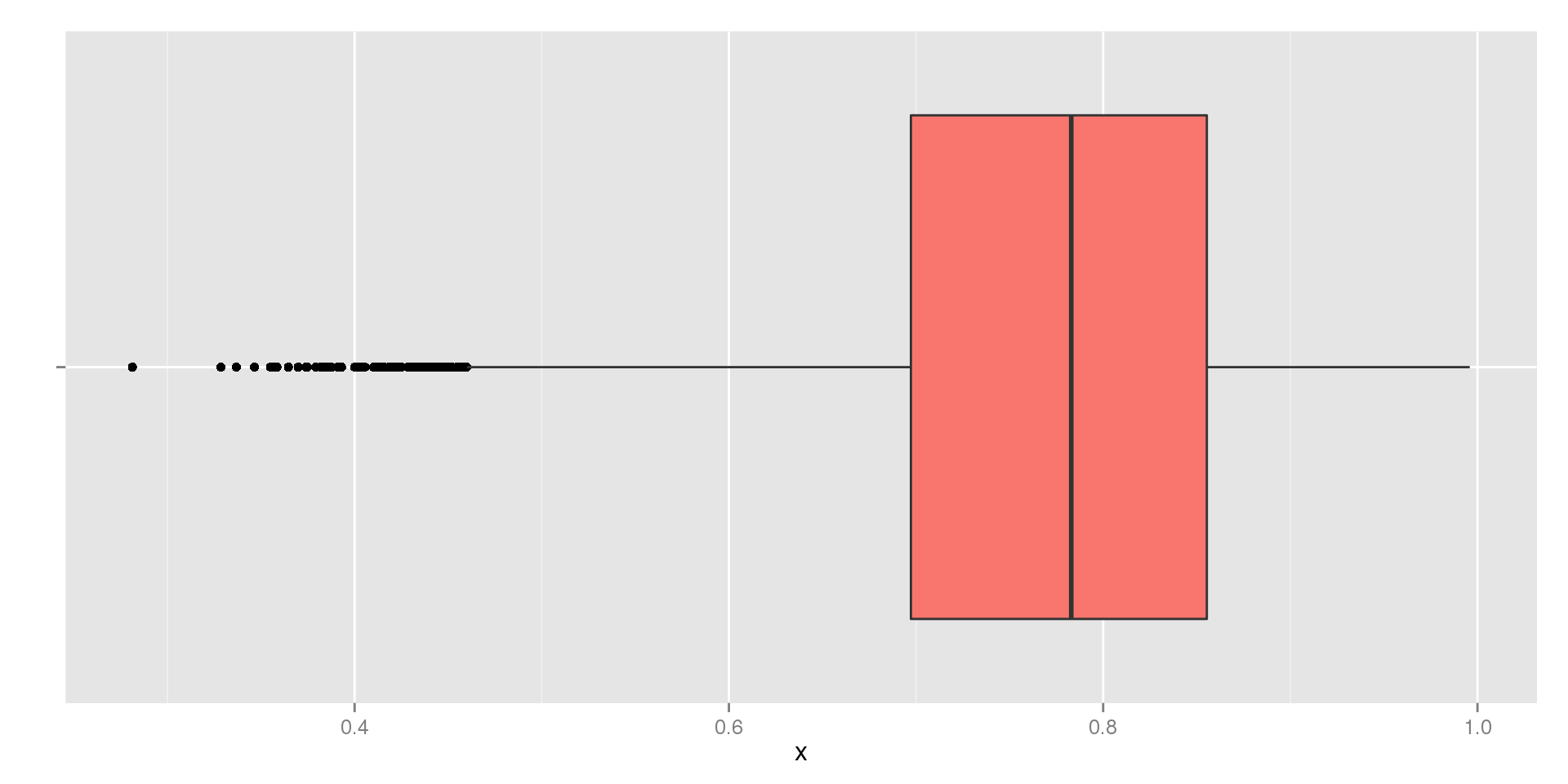## Symmetric Boxplot (Unimodal)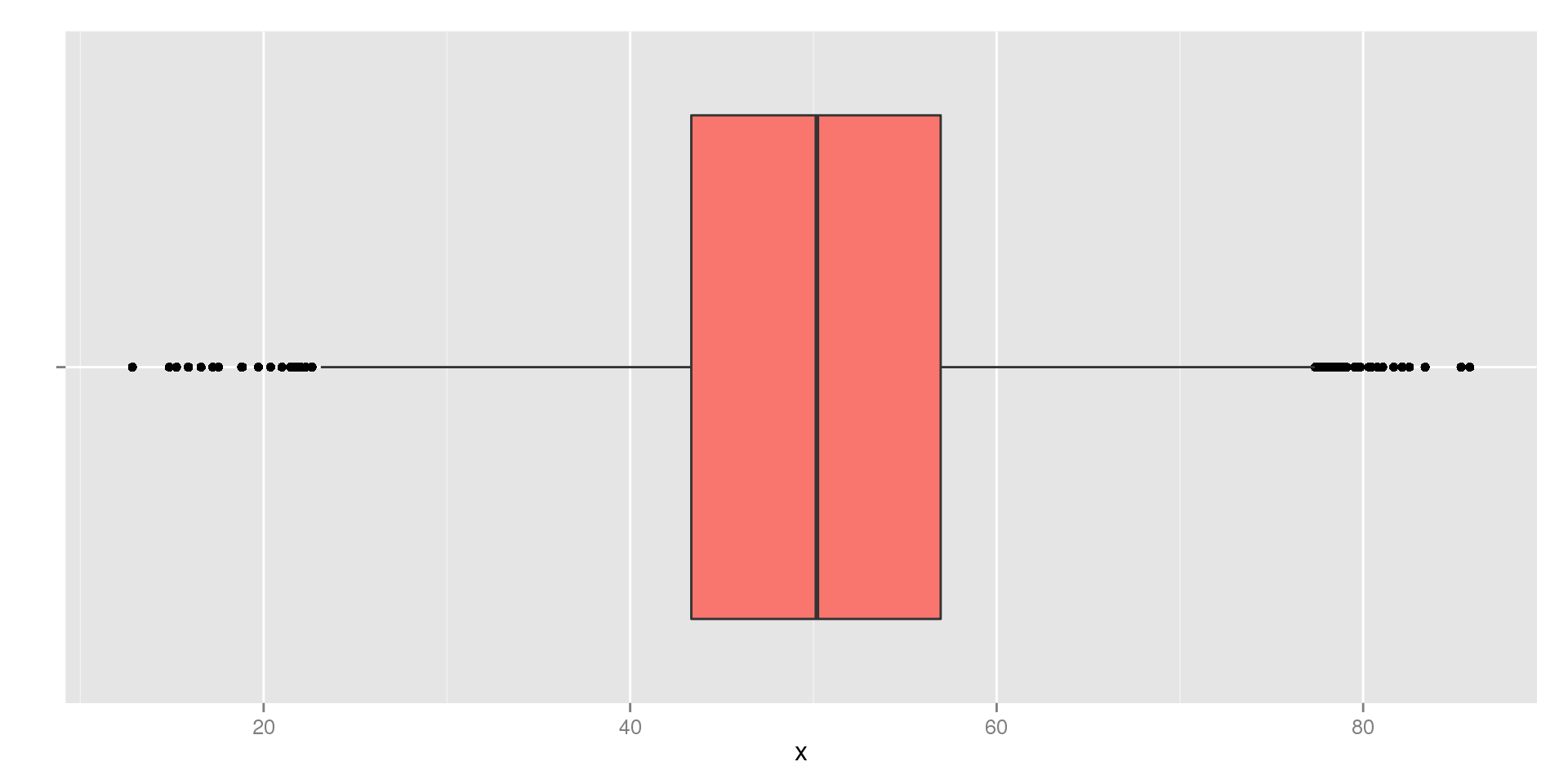## Symmetric Boxplot (Bimodal)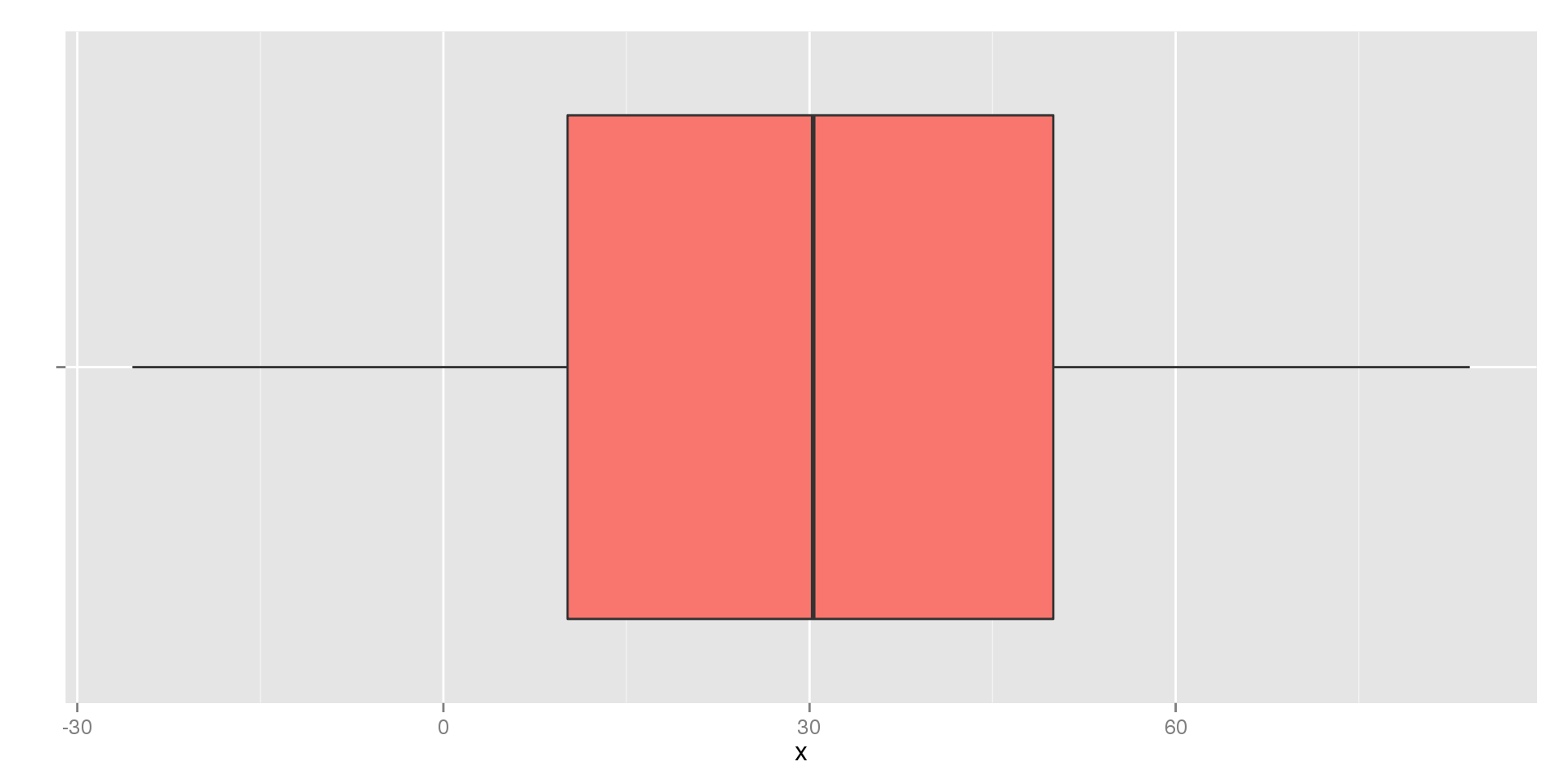## Interpreting a Boxplot

• The range of the data is the distance between the tips of the whiskers (or outliers) on either side
• The box represents the middle 50% of the data
• If the median is exactly in the center of the box, the data is symmetric
• Outliers can easily be seen
• The outliers, lengths of the whiskers, and position of the median tell us about the skew

Downsides:

• We lose some detail about the shape of the distribution (e.g., we can't see modes)
• Slight skew is harder to detect

## Measure of Center for Symmetric Distributions

The Median and IQR work for all distributions, symmetric or skewed. They are also very robust to outliers.

In the case of a symmetric distribution, we have a better alternative: the sample mean.

• The sample mean is what most people think of when they find an average
• As you've been doing for years, add up all the values and divide by how many there are

In more formal notation:

• $$\bar{x} = \frac{Total (Sum)}{n} = \frac{\sum x}{n}$$

If you picture the histogram as a physical object, the sample mean is the "balancing point"

## Calculating a Mean

Find the Mean of our data from earlier: $$1,\; 5,\; 7,\; 12,\; 18,\; 18,\; 25,\; 30$$

Finding the mean:

• $$\bar{x} = \frac{\sum x}{n}$$
• $$\bar{x} = \frac{1 + 5 + 7 + 12 + 18 + 18 + 25 + 30}{8}$$
• $$\bar{x} = \frac{116}{8}$$
• $$\bar{x} = 14.5$$

Recall that the median was $$15$$, so our two measures are fairly close.

## Means and Outliers

Now consider our data with an outlier: $$1,\; 5,\; 7,\; 12,\; 18,\; 18,\; 25,\; 60$$

Finding the mean:

• $$\bar{x} = \frac{\sum x}{n}$$
• $$\bar{x} = \frac{1 + 5 + 7 + 12 + 18 + 18 + 25 + 60}{8}$$
• $$\bar{x} = \frac{146}{8}$$
• $$\bar{x} = 18.5$$

Recall that the median of this data was still $$15$$.

• The mean is always pulled towards outliers

## The Mean vs. the Median

Image you have ten people in a room and want to know about their income. Nine of the people make $30 thousand per year, but one person makes$10 million.

In thousands of dollars, our data set looks like:

• $$30,\; 30,\; 30,\; 30,\; 30,\; 30,\; 30,\; 30,\; 30,\; 10000$$

What do the mean and median look like?

• Median: $30,000 • Mean:$1,027,000

If I asked you what the average person makes, which measure of center is better?

## Visualizing the Mean and Median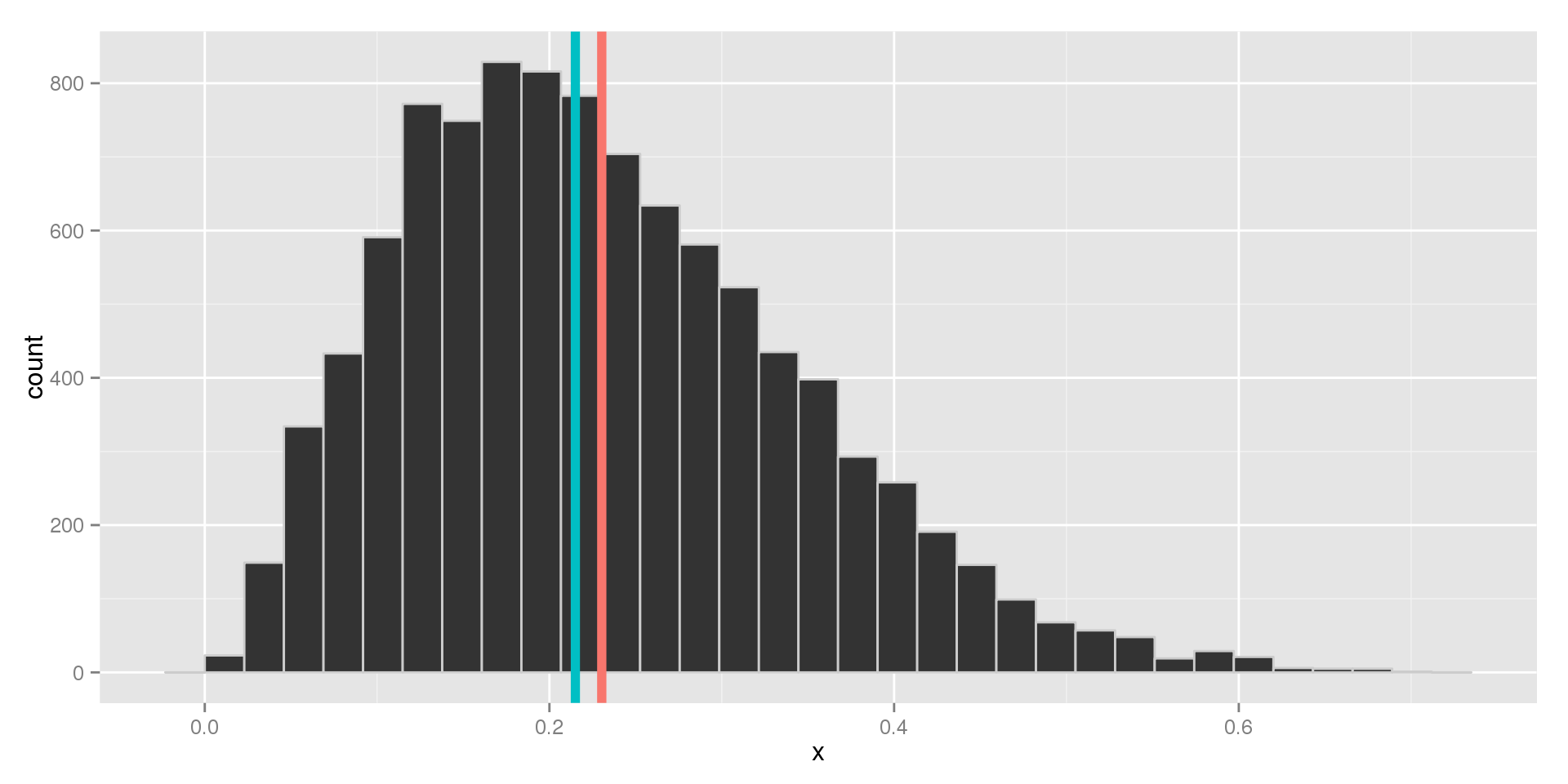Which is the mean and which is the median?

## Measure of Spread for Symmetric Data

Just like the IQR is based on the concept of the median, we have a measure of spread based on the same ideas as calculated the mean. We call the measure of spread based on the mean the standard deviation.

The standard deviation is related to a similar measure, the variance

• The variance and standard deviation tell us how far, on average, observations are from the mean
• They are essentially measures of uncertainty
• If the mean is a typical value, the variance tells us how far away from this we can generally expect to see observations

## The Variance

The formula for the variance is given by:

$s^2 = \frac{\sum (x - \bar{x})^2}{n-1}$

What does this formula mean?

• After finding the mean, subtract it from every value
• Square each difference to make it positive
• Add up all of the squared differences
• Divide by the number of observations minus one

So the variance gives is the average squared distance from the mean.

## The Standard Deviation

Note that the variance ends up being in the squared units of the variable. If $$x$$ was measured in feet, variance would be in square feet.

To correct the problem of having squared units, we take the square root of the variance. This measurement is called the standard deviation.

$s = \sqrt{s^2} = \sqrt{\frac{\sum (x - \bar{x})^2}{n-1}}$

• We interpret the standard deviation as the average distance of the points from the mean.
• Variances and standard deviations are always positive $$(s \ge 0)$$
• The closer together the values are, the smaller the standard deviation (and variance) will be

## The Standard Deviation and Outliers

$$1,\; 5,\; 7,\; 12,\; 18,\; 18,\; 25,\; 30$$

• $$s = 10.07$$

$$1,\; 5,\; 7,\; 12,\; 18,\; 18,\; 25,\; 60$$

• $$s = 18.62$$

As a rule:

• Making any value further away from the mean will increase $$s$$
• Making any value closer to the mean will decrease $$s$$

## Visualizing the Standard Deviation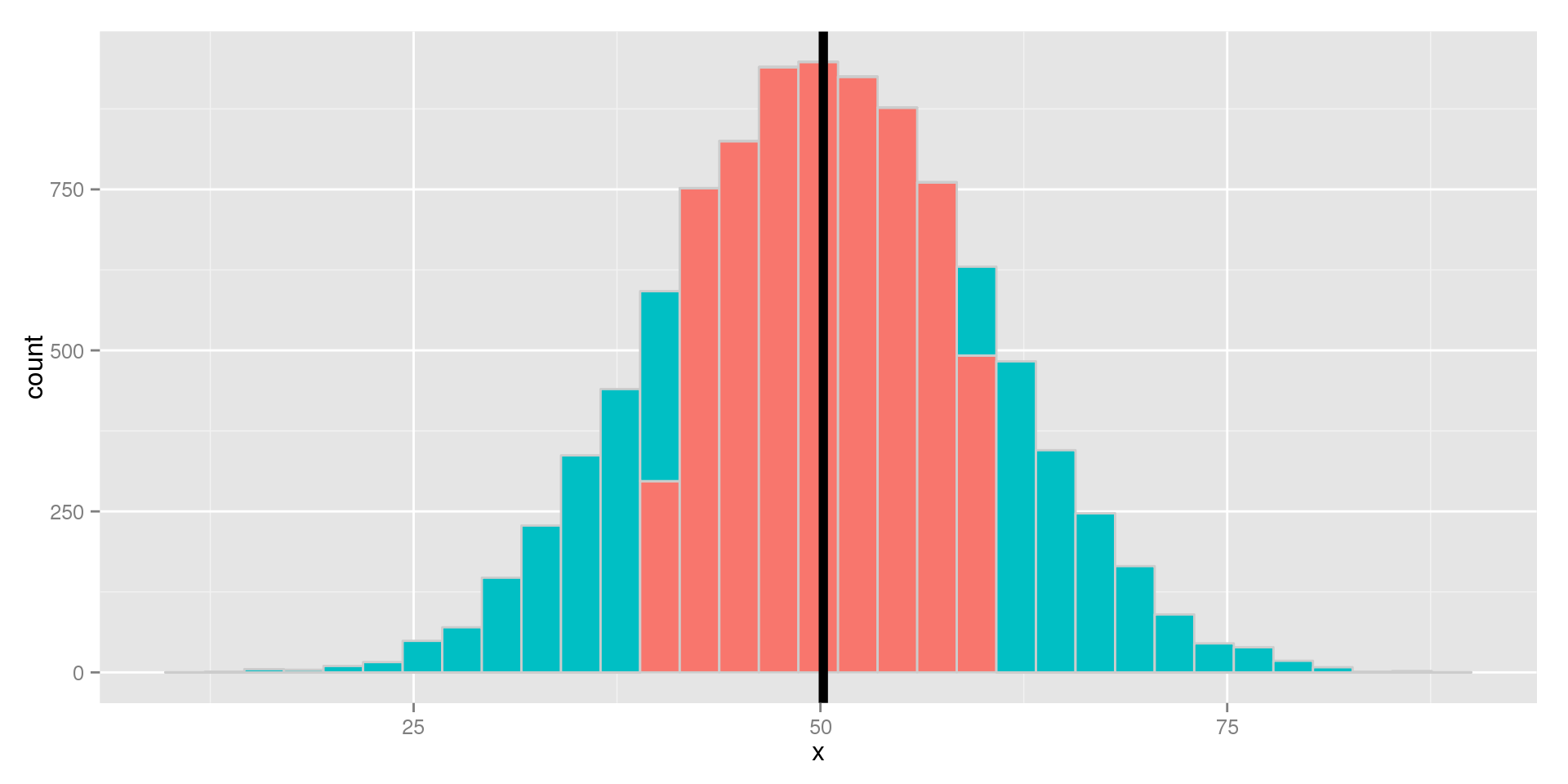## The Standard Deviation vs. the IQR

Recall the data set: $$1,\; 5,\; 7,\; 12,\; 18,\; 18,\; 25,\; 30$$

• The IQR is $$15.6$$. This means that the middle 50% of the observations were this far apart
• The Standard Deviation: $$s = 10.07$$, which means that the observations differ from the mean by $$10.07$$ units on average.

What about the version with an outlier? $$1,\; 5,\; 7,\; 12,\; 18,\; 18,\; 25,\; 60$$

• The IQR is still $$15.6$$
• $$s = 18.62$$

Earlier we said that the mean is worse than the median for skewed data. Since the standard deviation is calculated using the mean, if we throw out the mean we also need to throw out the standard deviation.

## Mean and Standard Deviation vs Median and IQR

So if both pairs of measures are good for symmetric data, and the median and IQR beat out the mean and standard deviation for skewed data, aren't the median and IQR always the better choices?

Not necessarily.

• The mean and standard deviation have properties that make them more useful
• The mean is faster to calculate (sorting numbers takes a relatively long time, even for computers, compared to adding them up)
• The median and IQR only take the order of the values into account, while the mean and standard deviation weigh large and small values differently

## Summary

• The shape of a distribution tells us how values are spread out across the individuals in our sample
• We can see the shape of a distribution using Histograms, Stem-and-Leaf Plots, and Boxplots
• The Five Number Summary gives us a quick overview of the distribution
• The Median and Mean tell us about typical values of the variable
• The Standard Deviation and IQR tell us about the spread of our variables
• The Median and IQR are robust to outliers and skew
• The Mean and Standard Deviation are generally preferred when the distribution is symmetric

## Important Notes

• These techniques are only for numeric variables (the mean area code is meaningless)
• Bar Charts and Histograms are not interchangeable
• Choosing the stems in a Stem-and-Leaf plot and number of bins in a Histogram are balancing acts
• Try to avoid rounding while calculating values, but don't report more decimal places than make sense
• Graph the data first, it will let you know which measures are appropriate to use